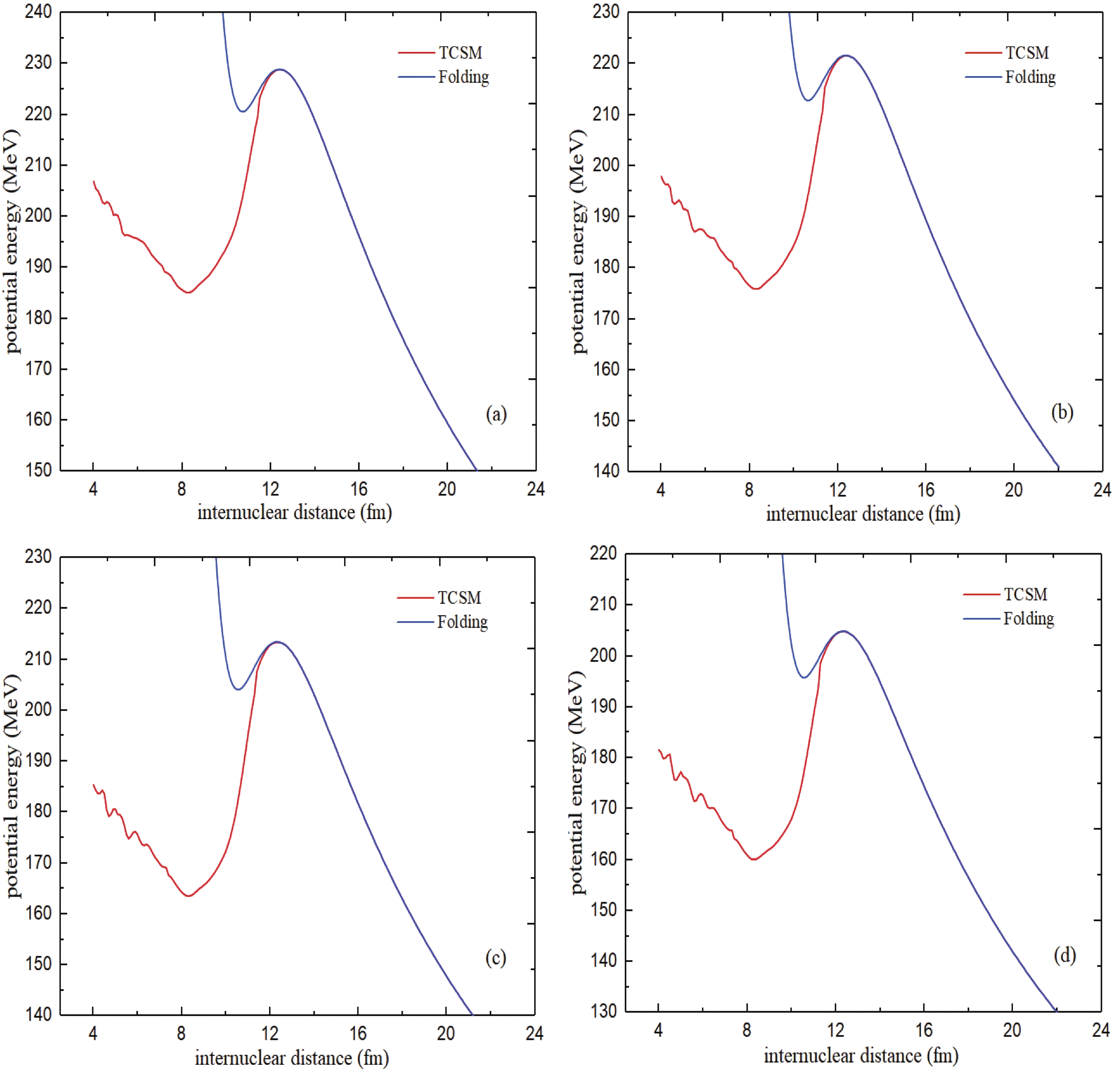# Study of the dinuclear system for 296119 superheavy compound nucleus in fusion reactions

• This investigation aims to find an appropriate dinuclear system for the formation of ${}^{296}$119 superheavy compound nucleus. By studying the driving potential and measuring the capture cross section of the reactions, the evolution of the dinuclear system can be understood. In this study, we obtained capture, fusion, and evaporation residue cross sections and survival probability at energies near the Coulomb barrier for four reactions, namely $^{45}$Sc + $^{251}$Cf, $^{42}$Ca + $^{254}$Es, $^{39}$K + $^{257}$Fm, and $^{38}$Ar + $^{258}$Md. Our calculations show that the reaction $^{38}$Ar + $^{258}$Md is a suitable choice for the formation of an element with 119 protons among the studied reactions from a theoretical viewpoint.
••Get Citation
J. Mohammadi and O. N. Ghodsi. Study of the dinuclear system for 296119 superheavy compound nucleus in the fusion reactions[J]. Chinese Physics C. doi: 10.1088/1674-1137/abe03e
J. Mohammadi and O. N. Ghodsi. Study of the dinuclear system for 296119 superheavy compound nucleus in the fusion reactions[J]. Chinese Physics C.Milestone
Received: 2020-10-30
Article Metric

Article Views(129)
PDF Downloads(25)
Cited by(0)
Policy on re-use
To reuse of subscription content published by CPC, the users need to request permission from CPC, unless the content was published under an Open Access license which automatically permits that type of reuse.
###### 通讯作者: 陈斌, bchen63@163.com
• 1.

沈阳化工大学材料科学与工程学院 沈阳 110142

Title:
Email:

## Study of the dinuclear system for 296119 superheavy compound nucleus in fusion reactions

###### Corresponding author: J. Mohammadi, J.Mohammadi@stu.umz.ac.ir
• Department of Physics, Faculty of Science, University of Mazandaran, P.O. Box 47415-416, Babolsar, Iran

Abstract: This investigation aims to find an appropriate dinuclear system for the formation of ${}^{296}$119 superheavy compound nucleus. By studying the driving potential and measuring the capture cross section of the reactions, the evolution of the dinuclear system can be understood. In this study, we obtained capture, fusion, and evaporation residue cross sections and survival probability at energies near the Coulomb barrier for four reactions, namely $^{45}$Sc + $^{251}$Cf, $^{42}$Ca + $^{254}$Es, $^{39}$K + $^{257}$Fm, and $^{38}$Ar + $^{258}$Md. Our calculations show that the reaction $^{38}$Ar + $^{258}$Md is a suitable choice for the formation of an element with 119 protons among the studied reactions from a theoretical viewpoint.

### HTMLII.   THEORETICAL FRAMEWORKIII.   RESULTS
Reference (42)
PDF查看关注分享

Top

### 目录/DownLoad:  Full-Size Img  PowerPoint